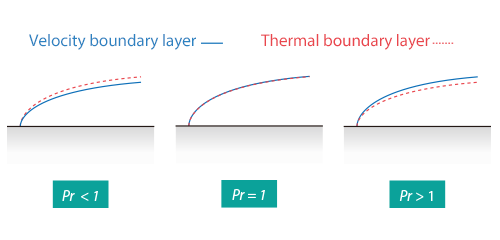#1. TOP
2. Resource Library
3. CAE Glossary
4. P-T index
5. Prandtl number

# Prandtl number

## Prandtl number

Prandtl number is a dimensionless number that represents the ratio of kinematic viscosity coefficient to thermal diffusion coefficient of a fluid.

It can be expressed with the following equation:where;
Pr : Prandtl number
: Kinematic viscosity coefficient [m2/s]
: Thermal diffusion coefficient [m2/s]
μ : Viscosity coefficient [Pa∙s]
C: Specific heat [J/(kg·K)]
: Thermal conductivity [W/(m·K)]

Prandtl number is a specific value for fluid and it represents the ratio of velocity boundary layer to thermal boundary layer. Prandtl number is named after a German physicist Ludwig Prandtl.